# NCERT Solutions for Class 6 Maths Chapter 2: Whole Numbers

In this page we have NCERT Solutions for Class 6 Maths Chapter 2: Whole Numbers. Hope you like them and do not forget to like , social share and comment at the end of the page.

## Exercise – 2.1

Question 1
Write the next three natural numbers after 10999.
Next three natural numbers after 10999 can be obtained by adding 1 to them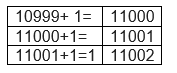So the numbers are 11000,11001,11002
Question 2:
Write the three whole numbers occurring just before 10001.
3 whole numbers just before 10001 can be obtained by subtracting 1 from them
 10001-1= 10000 10000-1= 9999 9999 -1 = 9998
So the numbers are 10000,9999,9998
Question 3
Which is the smallest whole number?
The smallest whole number is 0.
Question 4
How many whole numbers are there between 32 and 53?
Whole numbers between 32 and 53 can be find using subtracting the smaller number from bigger number, but it will include the highest number also, so subtracting 1 more. So it will be = 53 − 32 − 1 = 20
We can alternatively verify the answer by writing down all the whole number between them and counting them
33, 34, 35, 36, 37, 38, 39, 40, 41, 42, 43, 44, 45, 46, 47, 48, 49, 50, 51, 52
Question 5
Write the successor of:
(a) 2440701
(b) 100199
(c) 1099999
(d) 2345670
Successor are obtained by adding 1 to the number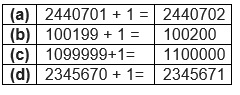Question 6
Write the predecessor of:
(a) 94
(b) 10000
(c) 208090
(d) 7654321
Predecessor is obtained by subtracting 1 from the Number
 a) 94 − 1 = 93 b) 10000 −1= 9999 c) 208090 − 1 = 208089 d) 7654321 − 1 = 7654320

Question 7
In each of the following pairs of numbers, state which whole number is on the left of the other number on the number line. Also write them with the appropriate sign (>, <) between them.
(a) 530, 503
(b) 370, 307
(c) 98765, 56789
(d) 9830415, 10023001
The Number line can be represented as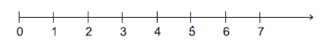The smaller is left side of the larger Number
(a) 530, 503
As 530 > 503,
503 is on the left side of 530 on the number line.
(b) 370, 307
As 370 > 307,
307 is on the left side of 370 on the number line.
(c) 98765, 56789
As 98765 > 56789,
56789 is on the left side of 98765 on the number line.
(d) 9830415, 10023001
Since 98, 30, 415 < 1, 00, 23, 001, 98,30,415 is on the left side of 1,00,23,001 on the number line.
Question 8
Which of the following statements are true (T) and which are false (F)?
(a) Zero is the smallest natural number.
(b) 400 is the predecessor of 399.
(c) Zero is the smallest whole number.
(d) 600 is the successor of 599.
(e) All natural numbers are whole numbers.
(f) All whole numbers are natural numbers.
(g) The predecessor of a two-digit number is never a single digit number.
(h) 1 is the smallest whole number.
(i) The natural number 1 has no predecessor.
(j) The whole number 1 has no predecessor.
(k) The whole number 13 lies between 11 and 12.
(l) The whole number 0 has no predecessor.
(m) The successor of a two-digit number is always a two-digit number.
(a) False, 0 is not a natural number. It is a smallest whole number
(b) False, as predecessor of 399 is 398 (399 − 1 = 398).
(c) True
(d) True, as 599 + 1 = 600
(e) True
(f) False, as 0 is a whole number but it is not a natural number.
(g) False. Example would be two-digit number 10 whose predecessor is 9.
(h) False, 0 is the smallest whole number.
(i) True, as 0 is the predecessor of 1 but it is not a natural number.
(j) False, as 0 is the predecessor of 1 and it is a whole number.
(k) False, 13 does not lie in between 11 and 12.
(l) True, predecessor of 0 is −1, which is not a whole number.
(m) False, as successor of 99 is 100.

## Exercise 2.2

Topic of Properties of Whole numbers is deleted from this chapter as per rationalized syllabus. You may ignore this exercise
Question 1
Find the sum by suitable rearrangement:
(a) $837 + 208 + 363$
(b) $1962 + 453 + 1538 + 647$
Rearranging the terms to make the addition simpler
(a) $837 + 208 + 363 = (837 + 363) + 208 = 1200 + 208 = 1408$
(b) $1962 + 453 + 1538 + 647 = (1962 + 1538) + (453 + 647) = 3500 + 1100 = 4600$
Question 2
Find the product by suitable rearrangement:
(a) $2 \times 1768 \times 50$
(b) $4 \times 166 \times 25$
(c) $8 \times 291 \times 125$
(d) $625 \times 279 \times 16$
(e) $285 \times 5 \times 60$
(f) $125 \times 40 \times 8 \times 25$
We can make the product calculation easier by making it multiples of 10,100 etc.
(a) $2 \times 1768 \times 50$
Here in this product, the product of 2 and 50 makes multiples of 100 which is easier to multiple with other number
= $2 \times 50 \times 1768 = 100 \times 1768 = 176800$
(b) $4 \times 166 \times 25$
Here in this product, the product of 4 and 25 makes multiples of 100 which is easier to multiple with other number
$= 4 \times 25 \times 166 = 100 \times 166 = 16600$
(c) $8 \times 291 \times 125$
Here in this product, the product of 8 and 125 makes multiples of 1000 which is easier to multiple with other number
$= 8 \times 125 \times 291 = 1000 \times 291 = 291000$
(d) $625 \times 279 \times 16= 625 \times 16 \times 279 = 10000 \times 279 = 2790000$
(e) $285 \times 5 \times 60= 285 \times 300 = 85500$
(f) $125 \times 40 \times 8 \times 25= 125 \times 8 \times 40 \times 25 = 1000 \times 1000 = 1000000$
Question 3
Find the value of the following:
(a) $297 \times 17 + 297 \times 3$
(b) $54279 \times 92 + 8 \times 54279$
(c) $81265 \times 169 - 81265 \times 69$
(d) $3845 \times 5 \times 782 + 769 \times 25 \times 218$
Here in these question, we can see a quantity common, so we can take it out of bracket and make the calculation easier
(a) $297 \times 17 + 297 \times 3$
Here 297 is common in both the term
$= 297 \times (17 + 3) = 297 \times 20 = 5940$
(b) $54279 \times 92 + 8 \times 54279$
Here 54279 is common in both the term
$= 54279 \times (92 + 8)$
$= 54279 \times 100 = 5427900$
(c) $81265 \times 169 - 81265 \times 69$
Here 81265 is common in both the term
$= 81265 \times (169 - 69) = 81265 \times 100 = 8126500$
(d) $3845 \times 5 \times 782 + 769 \times 25 \times 218$
Here we don’t common term as of now, so rearranging little bit as 769×5=3845
= 3845 × 5 × 782 + 769 × 5 × 5 × 218
= 3845 × 5 × 782 + 3845 × 5 × 218
= 3845 × 5 × (782 + 218)
= 19225 × 1000 = 19225000
Question 4:
Find the product using suitable properties.
(a) 738 × 103
(b) 854 × 102
(c) 258 × 1008
(d) 1005 × 168
We know that
 Distributive property If a, b and c are any two whole numbers, then a(b+c) = a×b + a×c.

(a) 738 × 103 = 738 × (100 + 3)
= 738 × 100 + 738 × 3 (Distributive property)
= 73800 + 2214
= 76014
(b) 854 × 102 = 854 × (100 + 2)
= 854 × 100 + 854 × 2 (Distributive property)
= 85400 + 1708 = 87108
(c) 258 × 1008 = 258 × (1000 + 8)
= 258 × 1000 + 258 × 8 (Distributive property)
= 258000 + 2064 = 260064
(d) 1005 × 168 = (1000 + 5) × 168
= 1000 × 168 + 5 × 168 (Distributive property)
= 168000 + 840 = 168840
Question 5
A taxi driver filled his car petrol tank with 40 litres of petrol on Monday. The next day, he filled the tank with 50 litres of petrol. If the petrol costs Rs 44 per litre, how much did he spend in all on petrol?
Fuel filled on Monday = 40 litres
Fuel filled on Tuesday = 50 litres
Total Petrol(Fuel) filled = (40 + 50) litres =90 Litres
Now Cost of 1L of petrol = Rs 44
Total money spent on Petrol = $44 \times 90$ = Rs 3960
Question 6
A vendor supplies 32 litres of milk to a hotel in the morning and 68 litres of milk in the evening. If the milk costs Rs 15 per litre, how much money is due to the vendor per day?
Milk supplied in the morning = 32 L
Milk supplied in the evening = 68 L
Total Quantity of milk given to hotel = (32 + 68) L
Cost of 1L of milk = Rs 15
Money due to vendor per day= $15 \times (32 + 68)$
$= 15 \times 100$=Rs 1500
Question 7
Match the following:
 (i) 425 × 136 = 425 × (6 + 30 +100) (a) Commutativity under multiplication. (ii) 2 × 49 × 50 = 2 × 50 × 49 (b) Commutativity under addition. (iii) 80 + 2005 + 20 = 80 + 20 + 2005 (c) Distributivity of multiplication over addition.

We Know that
 Closure Property If a and b are any two whole numbers, then $a + b$, $a \times b$ are also whole numbers. Commutative property If a and b are any two whole numbers, then $a+b = b+a$ and $a \times b = b \times a$ Associative property If a, b and c are any two whole numbers, then $(a+b)+c = a+(b+c)$ and $(a \times b) \times c = a \times (b \times c)$. Distributive property If a, b and c are any two whole numbers, then $a(b+c) = a \times b + a \times c$.

(i) 425 × 136 = 425 × (6 + 30 + 100)   which is Distributivity of multiplication over addition Hence, (c)
(ii) 2 × 49 × 50 = 2 × 50 × 49 which is Commutativity under multiplication Hence, (a)
(iii) 80 + 2005 + 20 = 80 + 20 + 2005 which is Commutativity under addition Hence, (b)

 (i) 425 × 136 = 425 × (6 + 30 +100) (c) Distributivity of multiplication over addition. (ii) 2 × 49 × 50 = 2 × 50 × 49 (a) Commutativity under multiplication. (iii) 80 + 2005 + 20 = 80 + 20 + 2005 (b) Commutativity under addition.

## Exercise 2.3

Topic of Properties of Whole numbers is deleted from this chapter as per rationalized syllabus. You may ignore this exercise
Question 1
Which of the following will not represent zero?
(a) $1 + 0$
(b) $0 \times 0$
c) $\frac {0}{2}$
d) $\frac {(10-10)}{2}$
 a) $1 + 0=1$ So it does not represent zero b) $0 \times 0=0$ So it represents zero c) $\frac {0}{2}=0$ So it represents zero d) $\frac {(10-10)}{2}=0$ So it represents zero
Question 2
If the product of two whole numbers is zero, can we say that one or both of them will be zero? Justify through examples.
If the product of 2 whole numbers is zero, then one of them is definitely zero.
We can take examples to justify it
For example,
0 × 11 = 0
968 × 0 = 0
0 × 0 = 0
If the product of 2 whole numbers is zero, then both of them may be zero.
Now if we take non zero numbers,
6 × 8 = 48
(Since numbers to be multiplied are not equal to zero, the result of the product will also be non-zero.)
Question 3
If the product of two whole numbers is 1, can we say that one of both of them will be 1? Justify through examples.
If the product of 2 numbers is 1, then both the numbers have to be equal to 1.
For example, 1 × 1 = 1
However, 1 × 9 = 9 or 12 × 1=12
Clearly, the product of two whole numbers will be 1 in the situation when both numbers to be multiplied are 1.
Question 4
Find using distributive property:
(a) $728 \times 101$
(b) $5437 \times 1001$
(c) $824 \times 25$
(d) $4275 \times 125$
(e) $504 \times 35$
We know that
 Distributive property If a, b and c are any two whole numbers, then $a(b+c) = a \times b + a \times c$.
(a) $728 \times 101= 728 \times (100 + 1)$
$= 728 \times 100 + 728 \times 1$
$= 72800 + 728 = 73528$
(b) $5437 \times 1001 = 5437 \times (1000 + 1)$
$= 5437 \times 1000 + 5437 \times 1$
$= 5437000 + 5437 = 5442437$
(c) $824 \times 25 = (825 -1) \times 25$
$= 825 \times 25 - 1 \times 25= (800 +25) \times 25 - 1 \times 25$
$= 800 \times 25 + 25 \times 25 - 1 \times 25$
$= 20000 + 600 = 20600$
(d) $4275 \times 125 = (4000 + 200 + 100 - 25) \times 125$
$= 4000 \times 125 + 200 \times 125 + 100 \times 125 - 25 \times 125$
$= 500000 + 25000 + 12500 - 3125$
= 534375
(e) $504 \times 35 = (500 + 4) \times 35$
$= 500 \times 35 + 4 \times 35$
$= 17500 + 140 = 17640$
Question 5
Study the pattern:
1 × 8 + 1 = 9
12 × 8 + 2 = 98
123 × 8 + 3 = 987
1234 × 8 + 4 = 9876
12345 × 8 + 5 = 98765
Write the next two steps. Can you say how the pattern works?
(Hint: 12345 = 11111 + 1111 + 111 + 11 + 1).
1 × 8 + 1 = 9
12 × 8 + 2 = 98
i.e. (11 + 1) × 8 + 2 = 11 × 8 + 1 × 8 + 2 = 88 + 8 + 2 = 98

123 × 8 + 3 = 987;
i.e. (111 + 11 + 1) × 8 + 3 = 111 × 8 + 11 × 8 + 1 × 8 + 2 = 888 + 88 + 8 + 3 = 987

1234 × 8 + 4 = 9876;
i.e. (1111 + 111 + 11 + 1) × 8 + 4 = 1111 × 8 + 111 × 8 + 11× 8 + 1 × 8 + 4
= 8888 + 888 + 88 + 8 + 4 = 9876
12345 × 8 + 5 = 98765
i.e. (11111 + 1111 + 111 + 11 + 1) × 8 + 5 = 11111 × 8 + 1111 × 8 + 111× 8 + 11 × 8 + 1 × 8 + 5
= 88888 + 8888 + 888 + 88 + 8 + 5 = 98765

Extending the pattern, we have
123456 × 8 + 6 =
(111111 + 11111 + 1111 + 111 + 11 + 1) × 8 + 5
= 111111 × 8 + 11111 × 8 + 1111 × 8 + 111× 8 + 11 × 8 + 1 × 8 + 5
= 888888 + 88888 + 8888 + 888 + 88 + 8 + 6 = 987654
1234567 × 8 + 7 =
(1111111 + 111111 + 11111 + 1111 + 111 + 11 + 1) × 8 + 7
= 1111111 × 8 + 111111 × 8 + 11111 × 8 + 1111 × 8 + 111× 8 + 11 × 8 + 1 × 8 + 7
= 8888888 + 888888 + 88888 + 8888 + 888 + 88 + 8 + 7 = 9876543

## Try These from NCERT work book

1. Write the predecessor and successor of
a.19
b.1997
c.12000
d.49
e.100000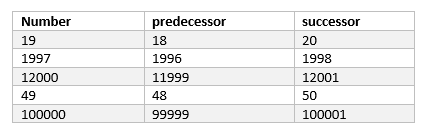2. Is there any natural number that has no predecessor?
1

3. Is there any natural number which has no successor? Is there a last natural number?
No, No

4. Are all natural numbers also whole numbers?
True

5. Are all whole numbers also natural numbers?
False

6. Which is the greatest whole number?
There is no largest whole number as every whole number has successor

7. Find using the number line.
a. 4 + 4
b. 2 + 6
c. 3 + 5
d. 1+6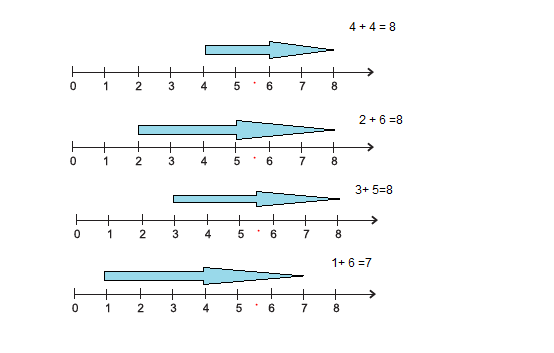8. Find using the number line.
a. 8 - 3
b. 6 - 2
c. 9 - 6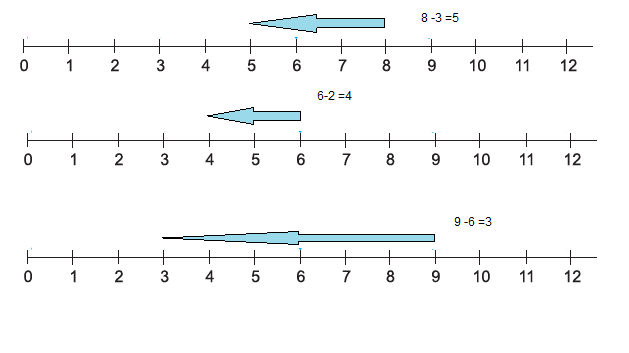9. Find using the number line
a. $2 \times 6$
b. $3 \times 3$
c. $4 \times 2$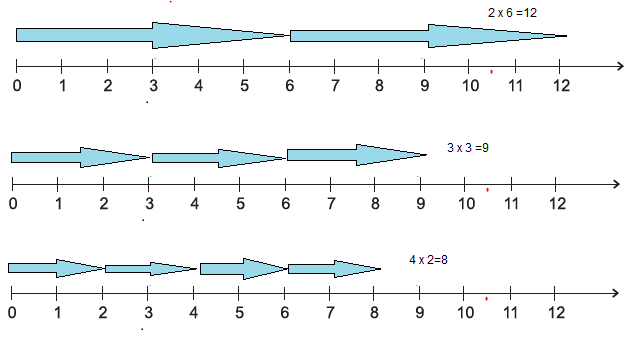10. Find
a. 7 + 18 + 13
b. 16 + 12 + 4
7 + 18 + 13= 7 + 13 + 18 = 20 + 18 = 38
16 + 12 + 4= 16 + 4 + 12= 20 + 12=32

11. Find
a. $25 \times 8358 \times 4$
b. $625 \times 3759 \times 8$
a. $25 \times 8358 \times 4 = 25 \times 4 \times 8358 = 100 \times 8358= 835800$
b. $625 \times 3759 \times 8 = 5 \times 125 \times 3759 \times 8 = 5 \times 125 \times 8 \times 3759= 5 \times 1000 \times 3759= 5 \times 3759000= 18795000$

12. Find using distributive property
a. $15 \times 68$
b. $17 \times 23$
c. $69 \times 78 + 22 \times 69$
a. $15 \times 68 =15 \times (60 + 8)= 900 + 120= 1020$
b. $17 \times 23 = 17 \times (20 + 3) = 340 + 51 = 391$

Play Game
You call a number from 1 to 10. Your friend now adds to this number any number from 1 to 10. Then it is your turn. You both play alternately. The winner is the one who reaches 100 first. If you always want to win the game, what will be your strategy or plan?
Strategy to win
You can surely win this game if your pre last chance end at 89 because your opponent can only maximum add 10, so it will be 99 ,Now you can add 1 and win the game. If he add 9, then it will be 98 ,now you can 2 to win the game

Now how to reach at 89.
This can be reached only if your early chance end at 78. Your opponent can maximum add 10,so it will be 88 and now you can add 1 to end at 89.

Now how to reach at 78.
This can be reached only if your early chance end at 67. Your opponent can maximum add 10,so it will be 77 and now you can add 1 to end at 78.

Now how to reach at 67.
This can be reached only if your early chance end at 56. Your opponent can maximum add 10,so it will be 66 and now you can add 1 to end at 67.

Now how to reach at 56.
This can be reached only if your early chance end at 45. Your opponent can maximum add 10,so it will be 55 and now you can add 1 to end at 56.

Now how to reach at 45.
This can be reached only if your early chance end at 34. Your opponent can maximum add 10,so it will be 44 and now you can add 1 to end at 45.

Now how to reach at 34.
This can be reached only if your early chance end at 23. Your opponent can maximum add 10,so it will be 33 and now you can add 1 to end at 34.

Now how to reach at 23.
This can be reached only if your early chance end at 12. Your opponent can maximum add 10,so it will be 22 and now you can add 1 to end at 23.

Now how to reach at 12.
This can be reached only if your early chance end at 1. Your opponent can maximum add 10,so it will be 11 and now you can add 1 to end at 12.
So, to win the game, your sum should add at 12,23,34,45,56,67,78,89 . We can see that it is like 1 ,1 +11, 1+11 + 11, ,1+11+11+11, 1+11+11+11+11 ....

So the trick is when you chance first make the no. 1 then your friends add "x" then you add (11-x) to reach at above sequence

• Notes
• Assignments
• NCERT Solutions### Practice Question

Question 1 What is $\frac {1}{2} + \frac {3}{4}$ ?
A)$\frac {5}{4}$
B)$\frac {1}{4}$
C)$1$
D)$\frac {4}{5}$
Question 2 Pinhole camera produces an ?
A)An erect and small image
B)an Inverted and small image
C)An inverted and enlarged image
D)None of the above